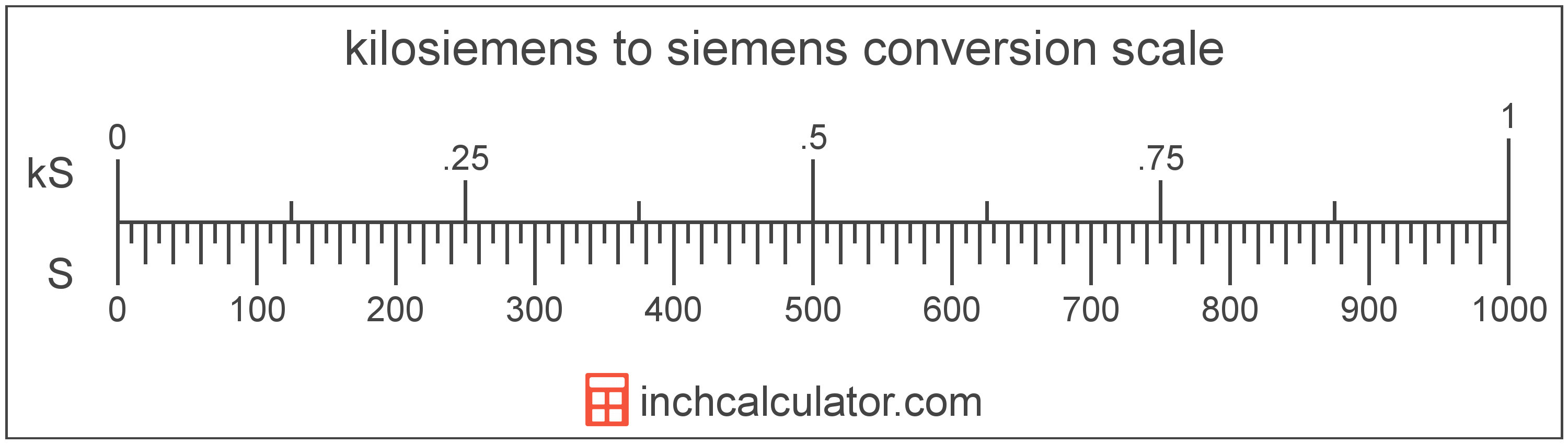# Kilosiemens to Siemens Conversion

Enter the electrical conductance in kilosiemens below to get the value converted to siemens.

Results in Siemens:1 kS = 1,000 S

## How to Convert Kilosiemens to SiemensTo convert a kilosiemens measurement to a siemens measurement, multiply the electrical conductance by the conversion ratio.

Since one kilosiemens is equal to 1,000 siemens, you can use this simple formula to convert:

siemens = kilosiemens × 1,000

The electrical conductance in siemens is equal to the kilosiemens multiplied by 1,000.

For example, here's how to convert 5 kilosiemens to siemens using the formula above.
5 kS = (5 × 1,000) = 5,000 S

### How Many Siemens are in a Kilosiemens?

There are 1,000 siemens in a kilosiemens, which is why we use this value in the formula above.

1 kS = 1,000 S

## Kilosiemens

The kilosiemens is the SI defined unit equal to the kilomho. One kilosiemens is the electrical conductance equal to 1,000 siemens, which are equal to one ampere per volt.

The kilosiemens is a multiple of the siemens, which is the SI derived unit for electrical conductance. In the metric system, "kilo" is the prefix for 103. Kilosiemens can be abbreviated as kS; for example, 1 kilosiemens can be written as 1 kS.

## Siemens

One siemens is the electrical conductance equal to an increase in current of one ampere for every increase in electrical potential difference of one volt. The siemens is the SI defined unit equal to the mho, and is the reciprocal of the resistance in ohms.

The siemens is the SI derived unit for electrical conductance in the metric system. Siemens can be abbreviated as S; for example, 1 siemens can be written as 1 S.

## Kilosiemens to Siemens Conversion Table

Kilosiemens measurements converted to siemens
Kilosiemens Siemens
0.001 kS 1 S
0.002 kS 2 S
0.003 kS 3 S
0.004 kS 4 S
0.005 kS 5 S
0.006 kS 6 S
0.007 kS 7 S
0.008 kS 8 S
0.009 kS 9 S
0.01 kS 10 S
0.02 kS 20 S
0.03 kS 30 S
0.04 kS 40 S
0.05 kS 50 S
0.06 kS 60 S
0.07 kS 70 S
0.08 kS 80 S
0.09 kS 90 S
0.1 kS 100 S
0.2 kS 200 S
0.3 kS 300 S
0.4 kS 400 S
0.5 kS 500 S
0.6 kS 600 S
0.7 kS 700 S
0.8 kS 800 S
0.9 kS 900 S
1 kS 1,000 S